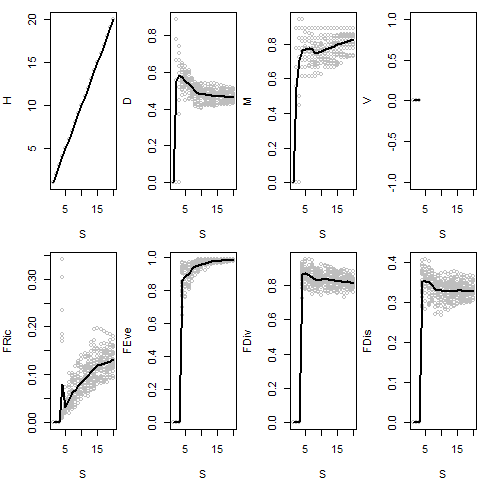# ecospace: Simulating Community Assembly and Ecological Diversification Using Ecospace Frameworks

#### 2020-06-13

ecospace is an R package that implements stochastic simulations of community assembly (ecological diversification) using customizable ecospace frameworks (functional trait spaces). Simulations model the ‘neutral’, ‘redundancy’, ‘partitioning’, and ‘expansion’ models of Bush and Novack-Gottshall (2012) and Novack-Gottshall (2016a,b). It provides a wrapper to calculate common ecological disparity and functional ecology statistical dynamics as a function of species richness. Functions are written so they will work in a parallel-computing environment.

The package also contains a sample data set, functional traits for Late Ordovician (Type Cincinnatian) fossil species from the Kope and Waynesville formations, from Novack-Gottshall (2016b).

## Create an ecospace framework (functional trait space)

Start by creating an ecospace framework with 9 characters: 3 as factors, 3 as ordered factors, and 3 as ordered numeric types. The framework is fully customizable, allowing users to use most character types, define unique character and state names, and constrain possible states (either by a set number of ‘multiple presences’ or by weighting the state according to a species pool).

library(ecospace)
nchar <- 9
ecospace <- create_ecospace(nchar = nchar, char.state = rep(3, nchar),
char.type = rep(c("factor", "ord.fac", "ord.num"), nchar / 3))

## Use ecospace framework to simulate a 50-species assemblage using the ‘neutral’ rule

In the ‘neutral’ model, all species have states chosen at random from the ecospace framework.

Smax <- 50
set.seed(3142)
neutral_sample <- neutral(Sseed = 5, Smax = Smax, ecospace = ecospace)
head(neutral_sample, 10)
##     char1  char2 char3  char4   char5 char6   char7   char8 char9
## 1  state2 state4   0.0 state8 state11   0.5 state15 state19   0.0
## 2  state3 state5   0.5 state8 state11   0.0 state16 state18   1.0
## 3  state2 state5   1.0 state8 state12   1.0 state16 state20   1.0
## 4  state3 state4   1.0 state8 state13   1.0 state17 state20   1.0
## 5  state2 state5   1.0 state9 state12   1.0 state15 state20   1.0
## 6  state2 state4   0.5 state8 state12   0.0 state16 state19   1.0
## 7  state2 state6   0.0 state8 state13   0.5 state15 state18   0.0
## 8  state1 state6   0.0 state8 state13   0.0 state17 state20   0.0
## 9  state2 state5   1.0 state8 state11   1.0 state15 state18   1.0
## 10 state2 state5   0.5 state8 state12   0.0 state16 state18   0.5

## Compare with assemblages built using the ‘redundancy’, ‘partitioning’, and ‘expansion’ rules

### Redundancy rules

The redundancy rules add species with traits redundant to those previously added. We will start the simulation by ‘seeding’ the assemblage with 5 species at random (before the rule starts).

set.seed(3142)
Sseed = 5
redund_sample <- redundancy(Sseed = Sseed, Smax = Smax, ecospace = ecospace)

Note that the number of functionally unique species will not change after the simulation begins in the default rule. Although there are 50 species, there are only 5 functionally unique entities.

unique(redund_sample)
##    char1  char2 char3  char4   char5 char6   char7   char8 char9
## 1 state2 state4   0.0 state8 state11   0.5 state15 state19     0
## 2 state3 state5   0.5 state8 state11   0.0 state16 state18     1
## 3 state2 state5   1.0 state8 state12   1.0 state16 state20     1
## 4 state3 state4   1.0 state8 state13   1.0 state17 state20     1
## 5 state2 state5   1.0 state9 state12   1.0 state15 state20     1

Relax the rule so that new species are on average 95% functionally identical to pre-existing ones:

set.seed(3142)
redund_sample2 <- redundancy(Sseed = Sseed, Smax = Smax,
ecospace = ecospace, strength = 0.95)

Plot both ‘redundancy’ assemblages (using PCA with Gower dissimilarity), showing order of assembly. Seed species in red, next 5 in black, remainder in gray. Notice the redundancy models produce an ecospace with discrete clusters of life habits.

library(FD, quietly = TRUE)
## This is vegan 2.5-6
pc <- prcomp(FD::gowdis(redund_sample))
plot(pc$x, type = "n", main = paste("Redundancy model,\n", Smax, "species")) text(pc$x[,1], pc$x[,2], labels = seq(Smax), col = c(rep("red", Sseed), rep("black", 5), rep("slategray", (Smax - Sseed - 5))), pch = c(rep(19, Sseed), rep(21, (Smax - Sseed))), cex = .8)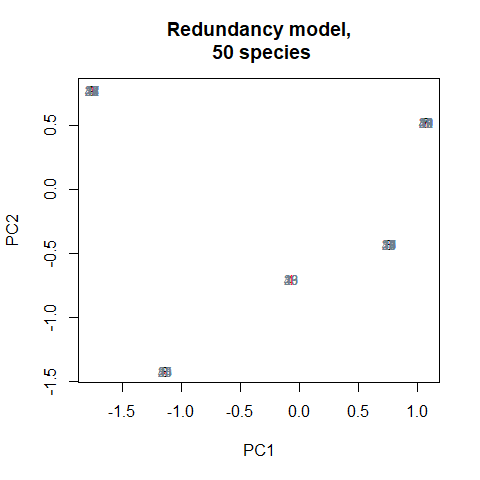pc.r <- prcomp(FD::gowdis(redund_sample2)) plot(pc.r$x, type = "n", main =
paste("Redundancy model (95% identical),\n", Smax, "species"))
text(pc.r$x[,1], pc.r$x[,2], labels = seq(Smax), col = c(rep("red",
Sseed), rep("black", 5), rep("slategray", (Smax - Sseed - 5))),
pch = c(rep(19, Sseed), rep(21, (Smax - Sseed))), cex = .8)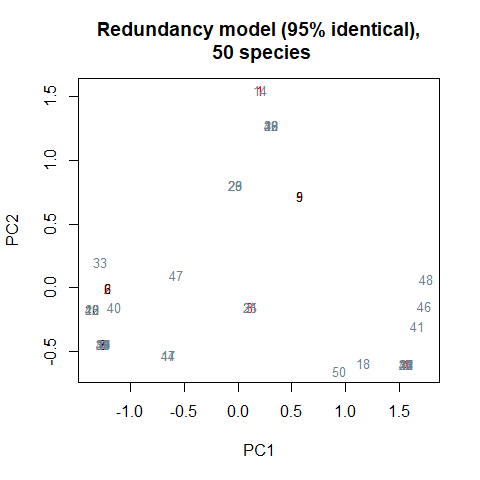### Partitioning rules

The partitioning rules add species with traits intermediate to those previously added. We will start the simulation by ‘seeding’ the assemblage with 5 species at random (before the rule starts).

The rules can be implemented in a “strict” (the default) version:

set.seed(3142)
Sseed = 5
partS_sample <- partitioning(Sseed = Sseed, Smax = Smax,
ecospace = ecospace)

Or in a “relaxed” version:

set.seed(3142)
Sseed = 5
partR_sample <- partitioning(Sseed = Sseed, Smax = Smax,
ecospace = ecospace, rule = "relaxed")

Plot both ‘partitioning’ assemblages, showing order of assembly. Notice both partitioning models produce gradients, with the ‘strict’ version having linear gradients and the ‘relaxed’ version filling the centroid.

pc.ps <- prcomp(FD::gowdis(partS_sample))
plot(pc.ps$x, type = "n", main = paste("'Strict' partitioning model,\n", Smax, "species")) text(pc.ps$x[,1], pc$x[,2], labels = seq(Smax), col = c(rep("red", Sseed), rep("black", 5), rep("slategray", (Smax - Sseed - 5))), pch = c(rep(19, Sseed), rep(21, (Smax - Sseed))), cex = .8)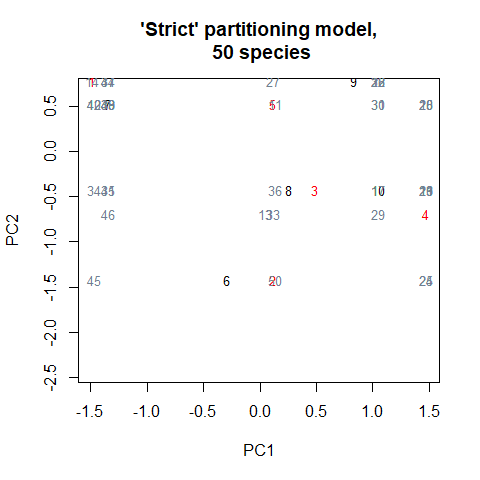pc.pr <- prcomp(FD::gowdis(partR_sample)) plot(pc.pr$x, type = "n",
main = paste("'Relaxed' partitioning model,\n", Smax, "species"))
text(pc.pr$x[,1], pc.pr$x[,2], labels = seq(Smax),
col = c(rep("red", Sseed), rep("black", 5),
rep("slategray", (Smax - Sseed - 5))),
pch = c(rep(19, Sseed), rep(21, (Smax - Sseed))), cex = .8)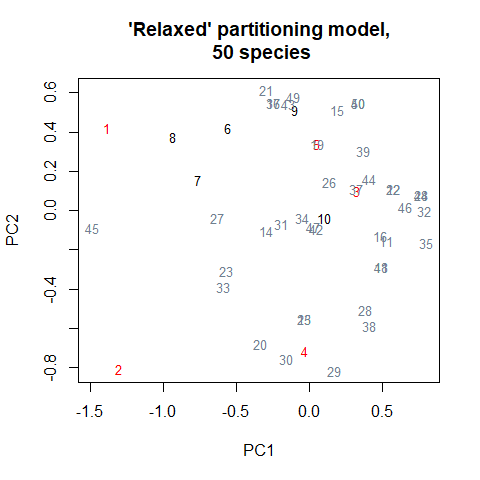### Expansion rules

The expansion rules add species with traits maximally different from those previously added. We will start the simulation by ‘seeding’ the assemblage with 5 species at random (before the rule starts).

set.seed(3142)
Sseed = 5
exp_sample <- expansion(Sseed = Sseed, Smax = Smax,
ecospace = ecospace)

Plot the assemblage, showing order of assembly. Notice how later species consistently expand the ecospace, exploring previously unexplored parts of the ecospace.

pc.e <- prcomp(FD::gowdis(exp_sample))
plot(pc.e$x, type = "n", main = paste("Expansion model,\n", Smax, "species")) text(pc.e$x[,1], pc$x[,2], labels = seq(Smax), col = c(rep("red", Sseed), rep("black", 5), rep("slategray", (Smax - Sseed - 5))), pch = c(rep(19, Sseed), rep(21, (Smax - Sseed))), cex = .8)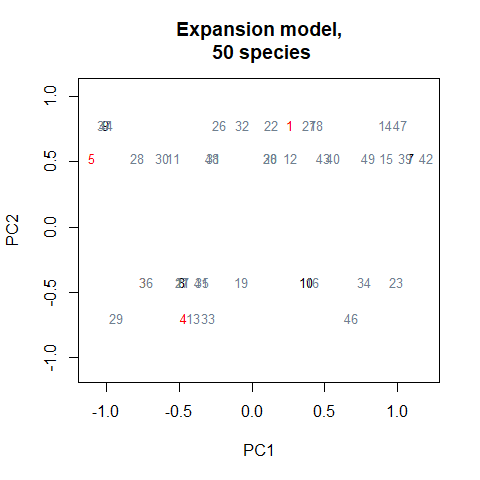### Visually comparing four rules It is instructive to compare the four models graphically. This is possible here because set.seed() was used when running each simulation, so they all share the same starting configurations. Plotting using vegan:metaMDS in two dimensions for improved visualization of simulation dynamics. library(vegan, quietly = TRUE) start <- neutral_sample[1:Sseed,] neu <- neutral_sample[(Sseed + 1):Smax,] red <- redund_sample2[(Sseed + 1):Smax,] par <- partR_sample[(Sseed + 1):Smax,] exp <- exp_sample[(Sseed + 1):Smax,] nmds.data <- rbind(start, neu, red, par, exp) all <- metaMDS(gowdis(nmds.data), zerodist = "add", k = 2, trymax = 10) ## Run 0 stress 0.2908926 ## Run 1 stress 0.3084162 ## Run 2 stress 0.2891901 ## ... New best solution ## ... Procrustes: rmse 0.02133373 max resid 0.1671276 ## Run 3 stress 0.2904407 ## Run 4 stress 0.2916602 ## Run 5 stress 0.3010061 ## Run 6 stress 0.3032821 ## Run 7 stress 0.2979624 ## Run 8 stress 0.293234 ## Run 9 stress 0.2979938 ## Run 10 stress 0.2978477 ## Run 11 stress 0.2934677 ## Run 12 stress 0.300761 ## Run 13 stress 0.2913372 ## Run 14 stress 0.2975214 ## Run 15 stress 0.2899913 ## Run 16 stress 0.3098921 ## Run 17 stress 0.3032887 ## Run 18 stress 0.2911977 ## Run 19 stress 0.2929413 ## Run 20 stress 0.3052993 ## *** No convergence -- monoMDS stopping criteria: ## 2: no. of iterations >= maxit ## 18: stress ratio > sratmax plot(all$points[,1], all$points[,2], col = c(rep("red", Sseed), rep("orange", nrow(neu)), rep("red", nrow(red)), rep("blue", nrow(par)), rep("purple", nrow(exp))), pch = c(rep(19, Sseed), rep(21, nrow(neu)), rep(22, nrow(red)), rep(23, nrow(par)), rep(24, nrow(exp))), main = paste("Combined models,\n", Smax, "species per model"), xlab = "Axis 1", ylab = "Axis 2", cex = 2, cex.lab = 1.5, lwd = 1) leg.txt <- c("seed", "neutral", "redundancy", "partitioning", "expansion") leg.col <- c("red", "orange", "red", "blue", "purple") leg.pch <- c(19, 21, 22, 23, 24) legend("topright", inset = .02, legend = leg.txt, pch = leg.pch, col = leg.col, cex = .75)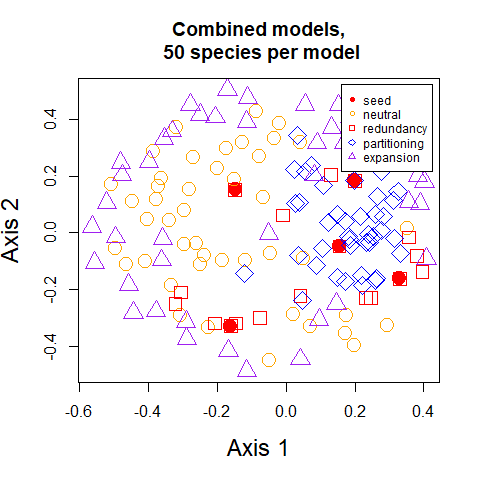## Calculate ecological disparity (functional diversity) metrics The package wraps around dbFD() in package FD to calculate common ecological disparity and functional diversity statistics. Statistics are calculated incrementally so dynamics can be understood as a function of species richness. See ?calc_metrics for explanation of each statistic. (Note: warnings are turned off in this vignette, caused by attempting to calculate the total variance (V) on factor characters.) Several users have requested changes to calc_metrics() to allow simple statistical calculation for the entire sample (instead of doing so incrementally). Starting with version 1.2.1, the argument increm = FALSE allows this functionality. # Using Smax = 10 here to illustrate calculation for first 25 species in neutral assemblage options(warn = -1) metrics <- calc_metrics(samples = neutral_sample, Smax = 10, Model = "Neutral") metrics ## Model Param S H D M V FRic FEve FDiv ## 1 Neutral 1 1 0.0000000 0.0000000 0 0.00000000 0.0000000 0.0000000 ## 2 Neutral 2 2 0.7777778 0.7777778 NA 0.00000000 0.0000000 0.0000000 ## 3 Neutral 3 3 0.5925926 0.6666667 NA 0.00000000 0.0000000 0.0000000 ## 4 Neutral 4 4 0.5555556 0.6666667 NA 0.01599345 0.8653846 0.8794282 ## 5 Neutral 5 5 0.5222222 0.6666667 NA 0.02895612 0.8389480 0.8245837 ## 6 Neutral 6 6 0.4697531 0.6481481 NA 0.03323198 0.9377974 0.7956808 ## 7 Neutral 7 7 0.4514991 0.7222222 NA 0.03003415 0.9315379 0.8180003 ## 8 Neutral 8 8 0.4821429 0.7222222 NA 0.06150281 0.9424795 0.8245066 ## 9 Neutral 9 9 0.4634774 0.8000000 NA 0.08701472 0.9698628 0.8276734 ## 10 Neutral 10 10 0.4402469 0.7962963 NA 0.08805487 0.9694741 0.8077878 ## FDis qual.FRic ## 1 0.0000000 0.0000000 ## 2 0.0000000 0.0000000 ## 3 0.0000000 0.0000000 ## 4 0.3401709 1.0000000 ## 5 0.3287315 1.0000000 ## 6 0.3023020 0.9574575 ## 7 0.2992515 0.9325871 ## 8 0.3247505 0.8671861 ## 9 0.3146638 0.7604866 ## 10 0.3010082 0.7339503 # Calculate statistics for just the entire sample options(warn = -1) metrics <- calc_metrics(samples = neutral_sample, increm = FALSE) metrics ## Model Param S H D M V FRic FEve FDiv ## 50 50 50 0.4604516 0.8950617 NA 0.1536291 0.9950255 0.8059989 ## FDis qual.FRic ## 50 0.3325312 0.2206635 The more typical use of calc_metrics() is to calculate statistics incrementally (which is the default behavior). # Using Smax = 10 here to illustrate calculation for first 10 species in neutral assemblage options(warn = -1) metrics <- calc_metrics(samples = neutral_sample, Smax = 10, Model = "Neutral", increm = TRUE) metrics ## Model Param S H D M V FRic FEve FDiv ## 1 Neutral 1 1 0.0000000 0.0000000 0 0.00000000 0.0000000 0.0000000 ## 2 Neutral 2 2 0.7777778 0.7777778 NA 0.00000000 0.0000000 0.0000000 ## 3 Neutral 3 3 0.5925926 0.6666667 NA 0.00000000 0.0000000 0.0000000 ## 4 Neutral 4 4 0.5555556 0.6666667 NA 0.01599345 0.8653846 0.8794282 ## 5 Neutral 5 5 0.5222222 0.6666667 NA 0.02895612 0.8389480 0.8245837 ## 6 Neutral 6 6 0.4697531 0.6481481 NA 0.03323198 0.9377974 0.7956808 ## 7 Neutral 7 7 0.4514991 0.7222222 NA 0.03003415 0.9315379 0.8180003 ## 8 Neutral 8 8 0.4821429 0.7222222 NA 0.06150281 0.9424795 0.8245066 ## 9 Neutral 9 9 0.4634774 0.8000000 NA 0.08701472 0.9698628 0.8276734 ## 10 Neutral 10 10 0.4402469 0.7962963 NA 0.08805487 0.9694741 0.8077878 ## FDis qual.FRic ## 1 0.0000000 0.0000000 ## 2 0.0000000 0.0000000 ## 3 0.0000000 0.0000000 ## 4 0.3401709 1.0000000 ## 5 0.3287315 1.0000000 ## 6 0.3023020 0.9574575 ## 7 0.2992515 0.9325871 ## 8 0.3247505 0.8671861 ## 9 0.3146638 0.7604866 ## 10 0.3010082 0.7339503 The functions are written so they can be run ‘in parallel’. Although not run here, the following provides an example of how this can be implemented using lapply(), here building 25 ‘neutral’ samples of 20 species each and then calculating disparity metrics on each. Note the code will take a few seconds to run to completion. nreps <- 1:25 # A sequence of the samples to be simulated n.samples <- lapply(X = nreps, FUN = neutral, Sseed = 3, Smax = 20, ecospace) # Calculate functional diversity metrics for simulated samples n.metrics <- lapply(X = nreps, FUN = calc_metrics, samples = n.samples, Model = "neutral", Param = "NA") # Combine lists together into a single dataframe (the function is new to this package, # but the newer 'rbindlist' function in 'data.table' package is even faster) all <- rbind_listdf(n.metrics) # Calculate mean dynamics across simulations means <- n.metrics[] for(n in 1:20) { means[n,4:11] <- apply(all[which(all$S == means$S[n]),4:11], 2, mean, na.rm = TRUE) } # Plot statistics as function of species richness, overlaying mean dynamics par(mfrow = c(2,4), mar = c(4, 4, 1, .3)) attach(all) plot(S, H, type = "p", cex = .75, col = "gray") lines(means$S, means$H, type = "l", lwd = 2) plot(S, D, type = "p", cex = .75, col = "gray") lines(means$S, means$D, type = "l", lwd = 2) plot(S, M, type = "p", cex = .75, col = "gray") lines(means$S, means$M, type = "l", lwd = 2) plot(S, V, type = "p", cex = .75, col = "gray") lines(means$S, means$V, type = "l", lwd = 2) plot(S, FRic, type = "p", cex = .75, col = "gray") lines(means$S, means$FRic, type = "l", lwd = 2) plot(S, FEve, type = "p", cex = .75, col = "gray") lines(means$S, means$FEve, type = "l", lwd = 2) plot(S, FDiv, type = "p", cex = .75, col = "gray") lines(means$S, means$FDiv, type = "l", lwd = 2) plot(S, FDis, type = "p", cex = .75, col = "gray") lines(means$S, means\$FDis, type = "l", lwd = 2)